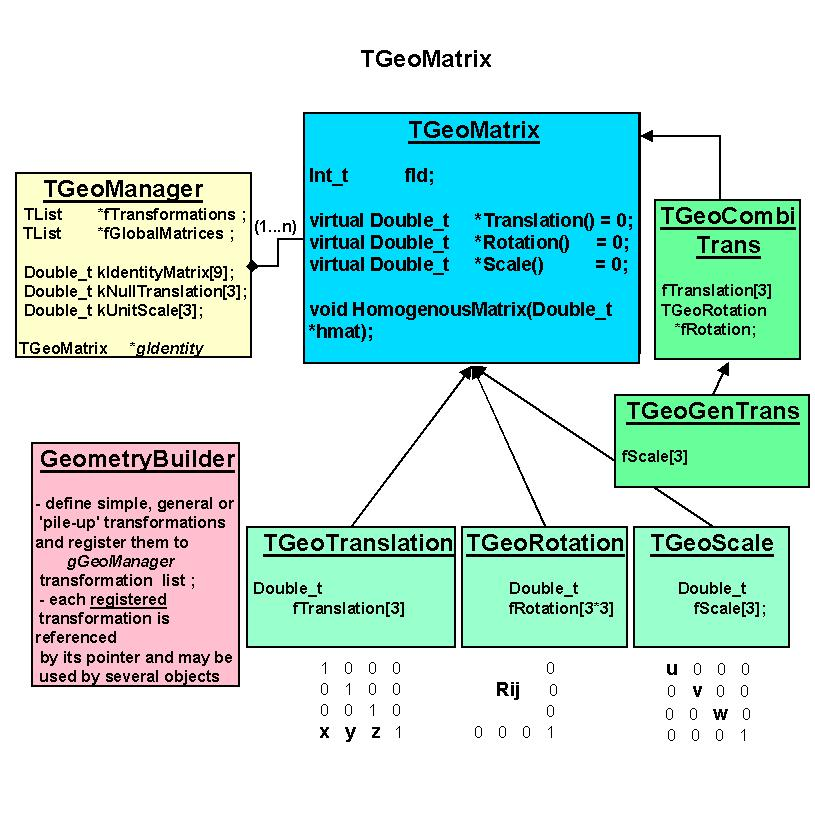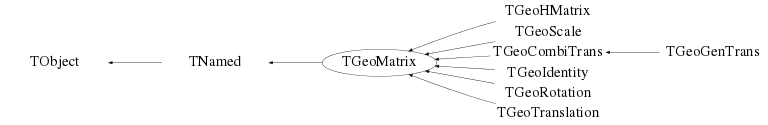# class TGeoMatrix: public TNamed

``` Geometrical transformation package.

All geometrical transformations handled by the modeller are provided as a
built-in package. This was designed to minimize memory requirements and
optimize performance of point/vector master-to-local and local-to-master
computation. We need to have in mind that a transformation in TGeo has 2
major use-cases. The first one is for defining the placement of a volume
with respect to its container reference frame. This frame will be called
'master' and the frame of the positioned volume - 'local'. If T is a
transformation used for positioning volume daughters, then:

MASTER = T * LOCAL

Therefore a local-to-master conversion will be performed by using T, while
a master-to-local by using its inverse. The second use case is the computation
of the global transformation of a given object in the geometry. Since the
geometry is built as 'volumes-inside-volumes', this global transformation
represent the pile-up of all local transformations in the corresponding
branch. The conversion from the global reference frame and the given object
is also called master-to-local, but it is handled by the manager class.
A general homogenous transformation is defined as a 4x4 matrix embeeding
a rotation, a translation and a scale. The advantage of this description
is that each basic transformation can be represented as a homogenous matrix,
composition being performed as simple matrix multiplication.
Rotation:                      Inverse rotation:
r11  r12  r13   0              r11  r21  r31   0
r21  r22  r23   0              r12  r22  r32   0
r31  r32  r33   0              r13  r23  r33   0
0    0    0    1               0    0    0    1

Translation:                   Inverse translation:
1    0    0    tx               1    0    0   -tx
0    1    0    ty               0    1    0   -ty
0    0    1    tz               0    0    1   -tz
0    0    0    1                0    0    0   1

Scale:                         Inverse scale:
sx   0    0    0              1/sx  0    0    0
0    sy   0    0               0   1/sy  0    0
0    0    sz   0               0    0   1/sz  0
0    0    0    1               0    0    0    1

where: rij are the 3x3 rotation matrix components,
tx, ty, tz are the translation components
sx, sy, sz are arbitrary scale constants on the eacks axis,

The disadvantage in using this approach is that computation for 4x4 matrices
is expensive. Even combining two translation would become a multiplication
of their corresponding matrices, which is quite an undesired effect. On the
other hand, it is not a good idea to store a translation as a block of 16
numbers. We have therefore chosen to implement each basic transformation type
as a class deriving from the same basic abstract class and handling its specific
data and point/vector transformation algorithms.

```
/**/
```
The base class TGeoMatrix defines abstract metods for:

- translation, rotation and scale getters. Every derived class stores only
its specific data, e.g. a translation stores an array of 3 doubles and a
rotation an array of 9. However, asking which is the rotation array of a
TGeoTranslation through the base TGeoMatrix interface is a legal operation.
The answer in this case is a pointer to a global constant array representing
an identity rotation.
Double_t *TGeoMatrix::GetTranslation()
Double_t *TGeoMatrix::GetRotation()
Double_t *TGeoMatrix::GetScale()

- MasterToLocal() and LocalToMaster() point and vector transformations :
void      TGeoMatrix::MasterToLocal(const Double_t *master, Double_t *local)
void      TGeoMatrix::LocalToMaster(const Double_t *local, Double_t *master)
void      TGeoMatrix::MasterToLocalVect(const Double_t *master, Double_t *local)
void      TGeoMatrix::LocalToMasterVect(const Double_t *local, Double_t *master)
These allow correct conversion also for reflections.
- Transformation type getters :
Bool_t    TGeoMatrix::IsIdentity()
Bool_t    TGeoMatrix::IsTranslation()
Bool_t    TGeoMatrix::IsRotation()
Bool_t    TGeoMatrix::IsScale()
Bool_t    TGeoMatrix::IsCombi() (translation + rotation)
Bool_t    TGeoMatrix::IsGeneral() (translation + rotation + scale)

Combinations of basic transformations are represented by specific classes
deriving from TGeoMatrix. In order to define a matrix as a combination of several
others, a special class TGeoHMatrix is provided. Here is an example of matrix
creation :

Matrix creation example:

root TGeoRotation r1,r2;
r1.SetAngles(90,0,30);        // rotation defined by Euler angles
r2.SetAngles(90,90,90,180,0,0); // rotation defined by GEANT3 angles
TGeoTranslation t1(-10,10,0);
TGeoTranslation t2(10,-10,5);
TGeoCombiTrans c1(t1,r1);
TGeoCombiTrans c2(t2,r2);
TGeoHMatrix h = c1 * c2; // composition is done via TGeoHMatrix class
root TGeoHMatrix *ph = new TGeoHMatrix(hm); // this is the one we want to
// use for positioning a volume
root ph->Print();

pVolume->AddNode(pVolDaughter,id,ph) // now ph is owned by the manager

Rule for matrix creation:
- unless explicitly used for positioning nodes (TGeoVolume::AddNode()) all
matrices deletion have to be managed by users. Matrices passed to geometry
have to be created by using new() operator and their deletion is done by
TGeoManager class.

Available geometrical transformations

1. TGeoTranslation - represent a (dx,dy,dz) translation. Data members:
Double_t fTranslation. Translations can be added/subtracted.
TGeoTranslation t1;
t1->SetTranslation(-5,10,4);
TGeoTranslation *t2 = new TGeoTranslation(4,3,10);
t2->Subtract(&t1);

2. Rotations - represent a pure rotation. Data members: Double_t fRotationMatrix[3*3].
Rotations can be defined either by Euler angles, either, by GEANT3 angles :
TGeoRotation *r1 = new TGeoRotation();
r1->SetAngles(phi, theta, psi); // all angles in degrees
This represent the composition of : first a rotation about Z axis with
angle phi, then a rotation with theta about the rotated X axis, and
finally a rotation with psi about the new Z axis.

r1->SetAngles(th1,phi1, th2,phi2, th3,phi3)
This is a rotation defined in GEANT3 style. Theta and phi are the spherical
angles of each axis of the rotated coordinate system with respect to the
initial one. This construction allows definition of malformed rotations,
e.g. not orthogonal. A check is performed and an error message is issued
in this case.

Specific utilities : determinant, inverse.

3. Scale transformations - represent a scale shrinking/enlargement. Data
members :Double_t fScale. Not fully implemented yet.

4. Combined transformations - represent a rotation folowed by a translation.
Data members: Double_t fTranslation, TGeoRotation *fRotation.
TGeoRotation *rot = new TGeoRotation("rot",10,20,30);
TGeoTranslation trans;

TGeoCombiTrans *c1 = new TGeoCombiTrans(trans, rot);
TGeoCombiTrans *c2 = new TGeoCombiTrans("somename",10,20,30,rot)

5. TGeoGenTrans - combined transformations including a scale. Not implemented.
6. TGeoIdentity - a generic singleton matrix representing a identity transformation
NOTE: identified by the global variable gGeoIdentity.

```

## Function Members (Methods)

This is an abstract class, constructors will not be documented.
Look at the header to check for available constructors.

public:
protected:
 virtual void TObject::DoError(int level, const char* location, const char* fmt, va_list va) const void TObject::MakeZombie() TGeoMatrix(const TGeoMatrix& other)

## Data Members

public:
 static TObject::(anonymous) TObject::kBitMask static TObject::EStatusBits TObject::kCanDelete static TObject::EStatusBits TObject::kCannotPick static TGeoMatrix::EGeoTransfTypes kGeoCombiTrans static TGeoMatrix::EGeoTransfTypes kGeoGenTrans static TGeoMatrix::EGeoTransfTypes kGeoIdentity static TGeoMatrix::EGeoTransfTypes kGeoMatrixOwned static TGeoMatrix::EGeoTransfTypes kGeoReflection static TGeoMatrix::EGeoTransfTypes kGeoRegistered static TGeoMatrix::EGeoTransfTypes kGeoRotation static TGeoMatrix::EGeoTransfTypes kGeoSavePrimitive static TGeoMatrix::EGeoTransfTypes kGeoScale static TGeoMatrix::EGeoTransfTypes kGeoShared static TGeoMatrix::EGeoTransfTypes kGeoTranslation static TObject::EStatusBits TObject::kHasUUID static TObject::EStatusBits TObject::kInvalidObject static TObject::(anonymous) TObject::kIsOnHeap static TObject::EStatusBits TObject::kIsReferenced static TObject::EStatusBits TObject::kMustCleanup static TObject::EStatusBits TObject::kNoContextMenu static TObject::(anonymous) TObject::kNotDeleted static TObject::EStatusBits TObject::kObjInCanvas static TObject::(anonymous) TObject::kOverwrite static TObject::(anonymous) TObject::kSingleKey static TObject::(anonymous) TObject::kWriteDelete static TObject::(anonymous) TObject::kZombie
protected:
 TString TNamed::fName object identifier TString TNamed::fTitle object title

## Class Charts## Function documentation

``` dummy constructor
```
TGeoMatrix(const TGeoMatrix& other)
``` copy constructor
```
TGeoMatrix(const char* name)
``` Constructor
```

``` Destructor
```
TGeoMatrix & operator*(const TGeoMatrix& right) const
``` Multiplication
```
``` Returns true if no rotation or the rotation is about Z axis
```
Int_t GetByteCount() const
``` Get total size in bytes of this
```
char * GetPointerName() const
``` Provide a pointer name containing uid.
```
void GetHomogenousMatrix(Double_t* hmat) const
``` The homogenous matrix associated with the transformation is used for
piling up's and visualization. A homogenous matrix is a 4*4 array
containing the translation, the rotation and the scale components

| R00*sx  R01    R02    dx |
| R10    R11*sy  R12    dy |
| R20     R21   R22*sz  dz |
|  0       0      0      1 |

where Rij is the rotation matrix, (sx, sy, sz) is the scale
transformation and (dx, dy, dz) is the translation.
```
void LocalToMaster(const Double_t* local, Double_t* master) const
``` convert a point by multiplying its column vector (x, y, z, 1) to matrix inverse
```
void LocalToMasterVect(const Double_t* local, Double_t* master) const
``` convert a vector by multiplying its column vector (x, y, z, 1) to matrix inverse
```
void LocalToMasterBomb(const Double_t* local, Double_t* master) const
``` convert a point by multiplying its column vector (x, y, z, 1) to matrix inverse
```
void MasterToLocal(const Double_t* master, Double_t* local) const
``` convert a point by multiplying its column vector (x, y, z, 1) to matrix
```
void MasterToLocalVect(const Double_t* master, Double_t* local) const
``` convert a point by multiplying its column vector (x, y, z, 1) to matrix
```
void MasterToLocalBomb(const Double_t* master, Double_t* local) const
``` convert a point by multiplying its column vector (x, y, z, 1) to matrix
```
void Normalize(Double_t* vect)
``` Normalize a vector.
```
void Print(Option_t* option = "") const
``` print the matrix in 4x4 format
```
void ReflectX(Bool_t leftside, Bool_t rotonly = kFALSE)
``` Multiply by a reflection respect to YZ.
```
void ReflectY(Bool_t leftside, Bool_t rotonly = kFALSE)
``` Multiply by a reflection respect to ZX.
```
void ReflectZ(Bool_t leftside, Bool_t rotonly = kFALSE)
``` Multiply by a reflection respect to XY.
```
void RegisterYourself()
``` Register the matrix in the current manager, which will become the owner.
```
void SetDefaultName()
``` If no name was supplied in the ctor, the type of transformation is checked.
A letter will be prepended to the name :
t - translation
r - rotation
s - scale
c - combi (translation + rotation)
g - general (tr+rot+scale)
The index of the transformation in gGeoManager list of transformations will
be appended.
```
TGeoMatrix& operator=(const TGeoMatrix& matrix)
``` Preventing warnings with -Weffc++ in GCC since the behaviour of operator * was chosen so by design.
```
Bool_t IsIdentity() const
`{return !TestBit(kGeoGenTrans);}`
Bool_t IsTranslation() const
`{return TestBit(kGeoTranslation);}`
Bool_t IsRotation() const
`{return TestBit(kGeoRotation);}`
Bool_t IsReflection() const
`{return TestBit(kGeoReflection);}`
Bool_t IsScale() const
`{return TestBit(kGeoScale);}`
Bool_t IsShared() const
`{return TestBit(kGeoShared);}`
Bool_t IsCombi() const
Bool_t IsGeneral() const
Bool_t IsRegistered() const
`{return TestBit(kGeoRegistered);}`
const Double_t * GetTranslation() const
const Double_t * GetRotationMatrix() const
const Double_t * GetScale() const
TGeoMatrix& Inverse() const
TGeoMatrix * MakeClone() const
void RotateX(Double_t )
`{}`
void RotateY(Double_t )
`{}`
void RotateZ(Double_t )
`{}`
void SetDx(Double_t )
`{}`
void SetDy(Double_t )
`{}`
void SetDz(Double_t )
`{}`
void SetShared(Bool_t flag = kTRUE)
`{SetBit(kGeoShared, flag);}`